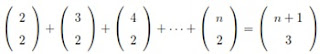1. Use Mathematical induction to show that if n > 1 then (Max score: 25)2. Prove that no integer in the following sequence is a perfect square: (Max score: 25)
11; 111; 1111; 11111;
[Hint: A typical term 111 111 can be written as 111 111 = 111 108+3 = 4k+3.]

3. Determine all solutions in the positive integers of the Diophantine equation
158x  57y = 7;
(Max score: 25)

4. Show that the only prime p for which 3p + 1 is a perfect square is p = 5.
(Max score: 25)